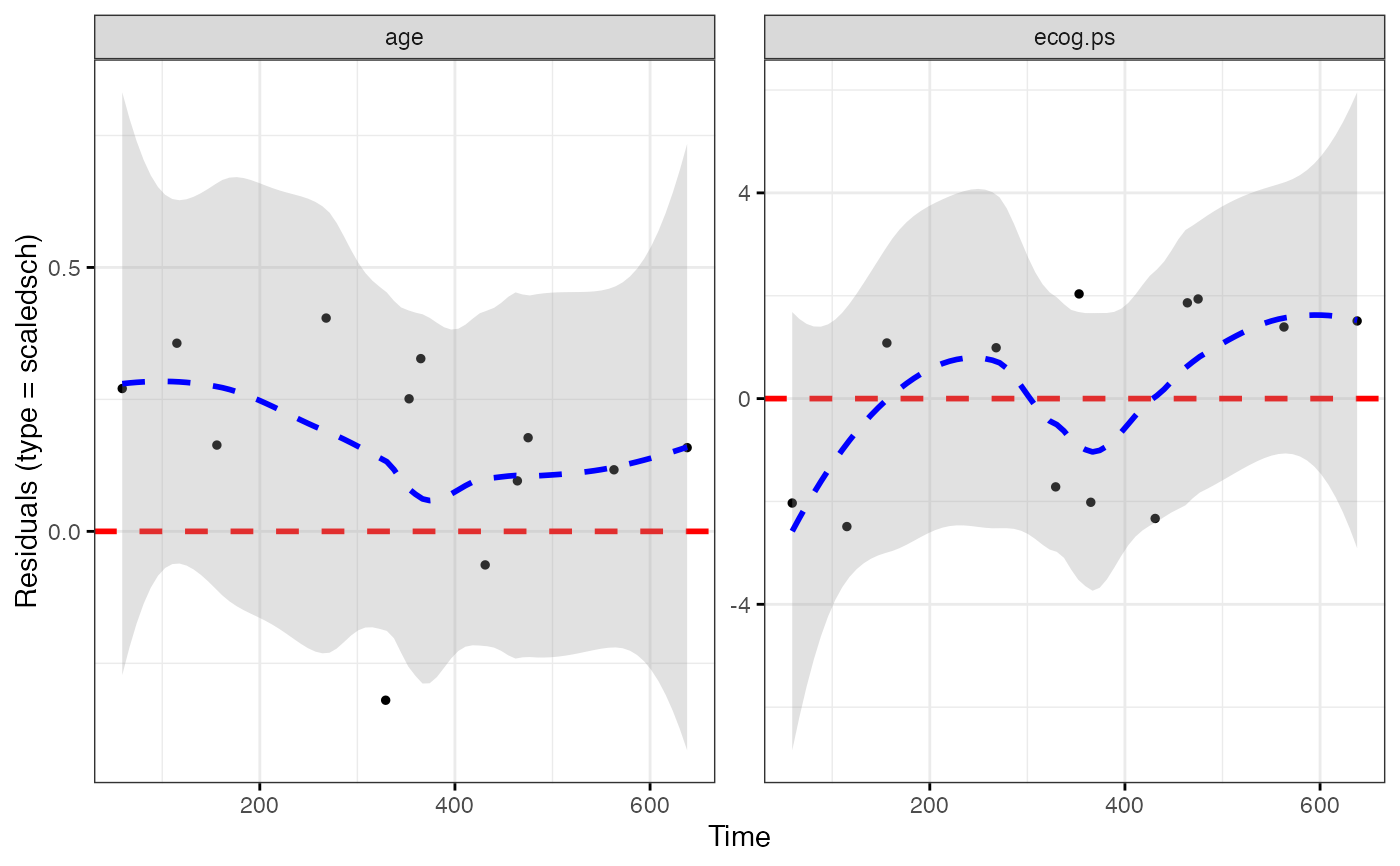Displays diagnostics graphs presenting goodness of Cox Proportional Hazards Model fit, that can be calculated with coxph function.

ggcoxdiagnostics(
fit,
type = c("martingale", "deviance", "score", "schoenfeld", "dfbeta", "dfbetas",
"scaledsch", "partial"),
...,
linear.predictions = type %in% c("martingale", "deviance"),
ox.scale = ifelse(linear.predictions, "linear.predictions", "observation.id"),
hline = TRUE,
sline = TRUE,
sline.se = TRUE,
hline.col = "red",
hline.size = 1,
hline.alpha = 1,
hline.yintercept = 0,
hline.lty = "dashed",
sline.col = "blue",
sline.size = 1,
sline.alpha = 0.3,
sline.lty = "dashed",
point.col = "black",
point.size = 1,
point.shape = 19,
point.alpha = 1,
title = NULL,
subtitle = NULL,
caption = NULL,
ggtheme = ggplot2::theme_bw()
)

## Arguments

fit an object of class coxph.object - created with coxph function. the type of residuals to present on Y axis of a diagnostic plot. The same as in residuals.coxph: character string indicating the type of residual desired. Possible values are "martingale", "deviance", "score", "schoenfeld", "dfbeta", "dfbetas" and "scaledsch". Only enough of the string to determine a unique match is required. further arguments passed to residuals.coxph or to the function ggpar for customizing the plot. (deprecated, see ox.scale) a logical value indicating whether to show linear predictions for observations (TRUE) or just indexed of observations (FALSE) on X axis. one value from c("linear.predictions", "observation.id", "time"). It defines what will be presented on OX scale. Possible values: y hat for "linear.predictions", Id of an observation for "observation.id" or Time for "time". a logical - should the horizontal line be added to highlight the Y=0 level. a logical - should the smooth line be added to highlight the local average for residuals. color, size, linetype, visibility and Y-axis coordinate to be used for geom_hline. Used only when hline = TRUE. color, size, linetype and visibility to be used for geom_smooth. Used only when sline = TRUE. color, size, shape and visibility to be used for points. main title, subtitle and caption. function, ggplot2 theme name. Default value is ggplot2::theme_bw(). Allowed values include ggplot2 official themes: see theme.

## Value

Returns an object of class ggplot.

## Functions

• ggcoxdiagnostics: Diagnostic Plots for Cox Proportional Hazards Model with ggplot2

## Examples


library(survival)
coxph.fit2 <- coxph(Surv(futime, fustat) ~ age + ecog.ps, data=ovarian)
ggcoxdiagnostics(coxph.fit2, type = "deviance")#> geom_smooth() using formula 'y ~ x'ggcoxdiagnostics(coxph.fit2, type = "schoenfeld", title = "Diagnostic plot")#> geom_smooth() using formula 'y ~ x'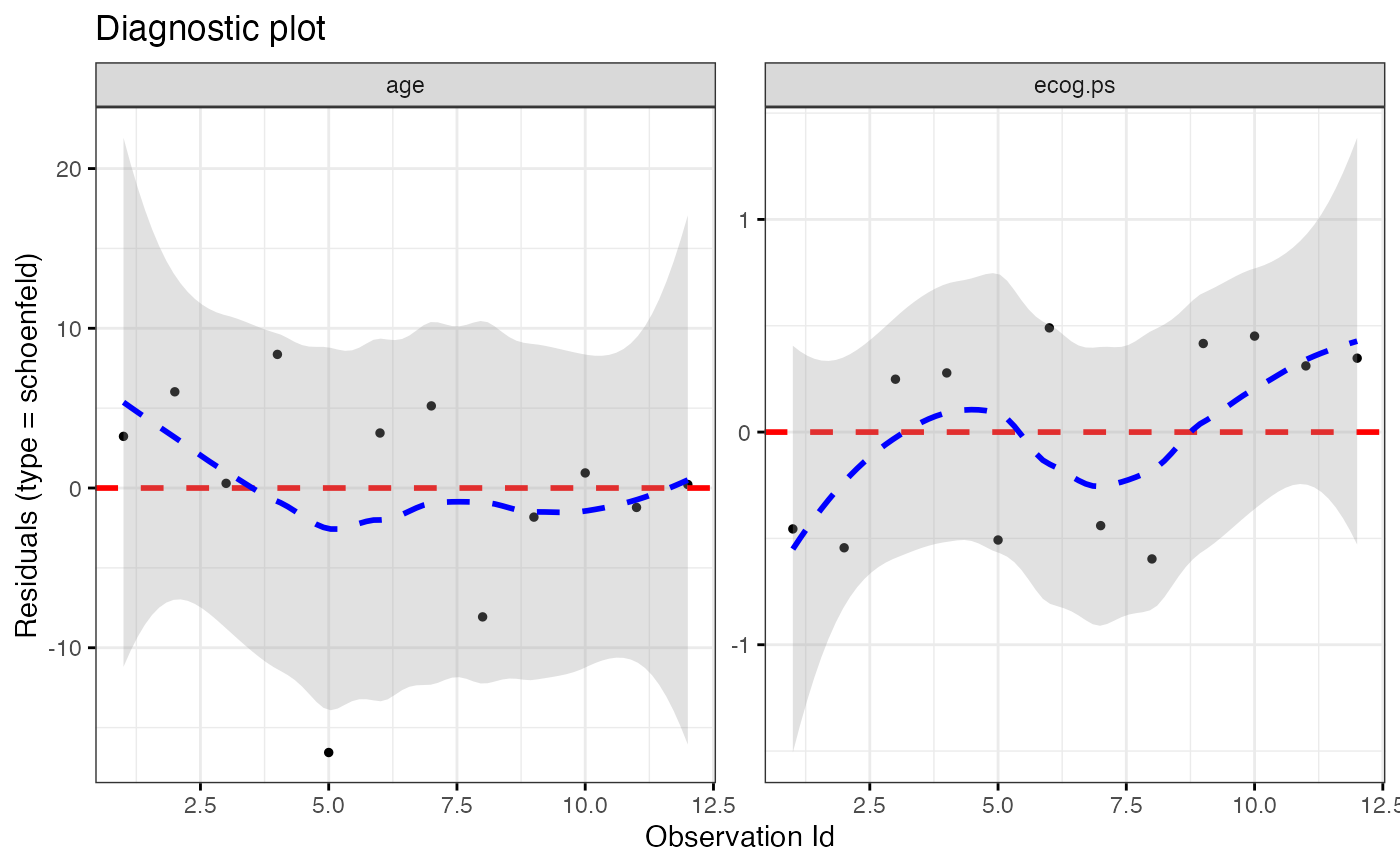ggcoxdiagnostics(coxph.fit2, type = "deviance", ox.scale = "time")#> Warning: ox.scale='time' works only with type=schoenfeld/scaledsch#> geom_smooth() using formula 'y ~ x'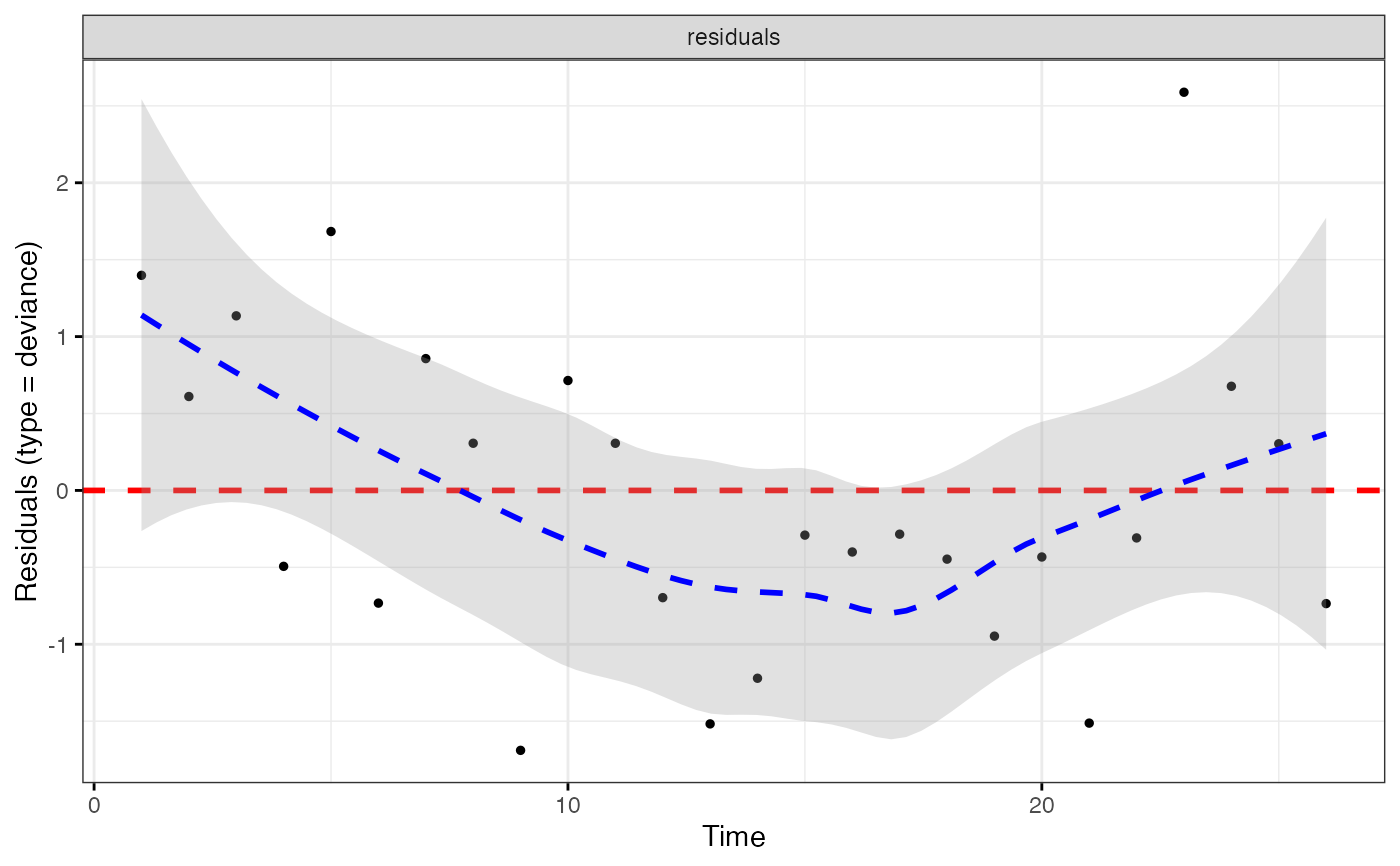ggcoxdiagnostics(coxph.fit2, type = "schoenfeld", ox.scale = "time",
title = "Diagnostic plot", subtitle = "Data comes from survey XYZ",
font.subtitle = 9)#> geom_smooth() using formula 'y ~ x'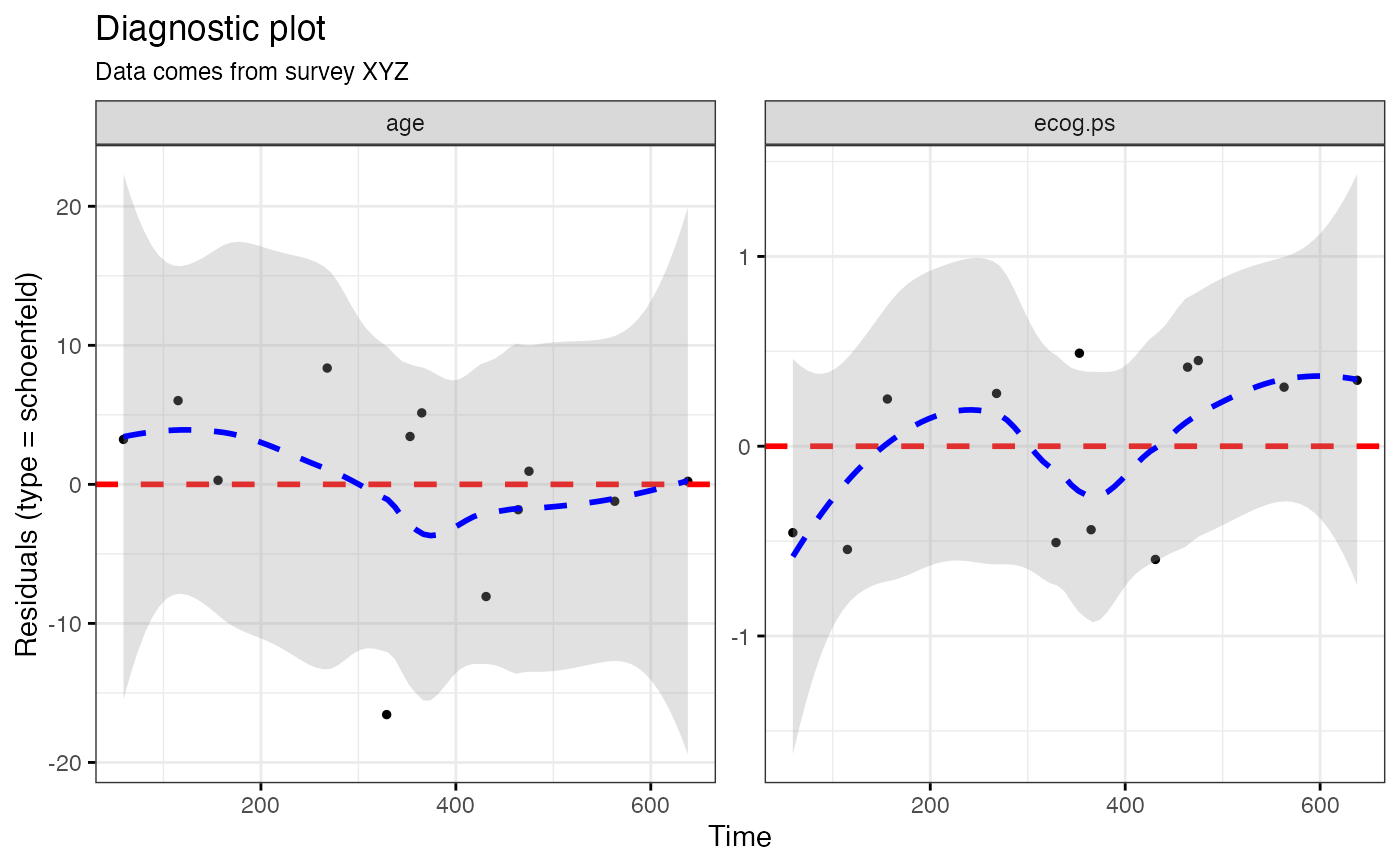ggcoxdiagnostics(coxph.fit2, type = "deviance", ox.scale = "linear.predictions",
caption = "Code is available here - link", font.caption = 10)#> geom_smooth() using formula 'y ~ x'ggcoxdiagnostics(coxph.fit2, type = "schoenfeld", ox.scale = "observation.id")#> geom_smooth() using formula 'y ~ x'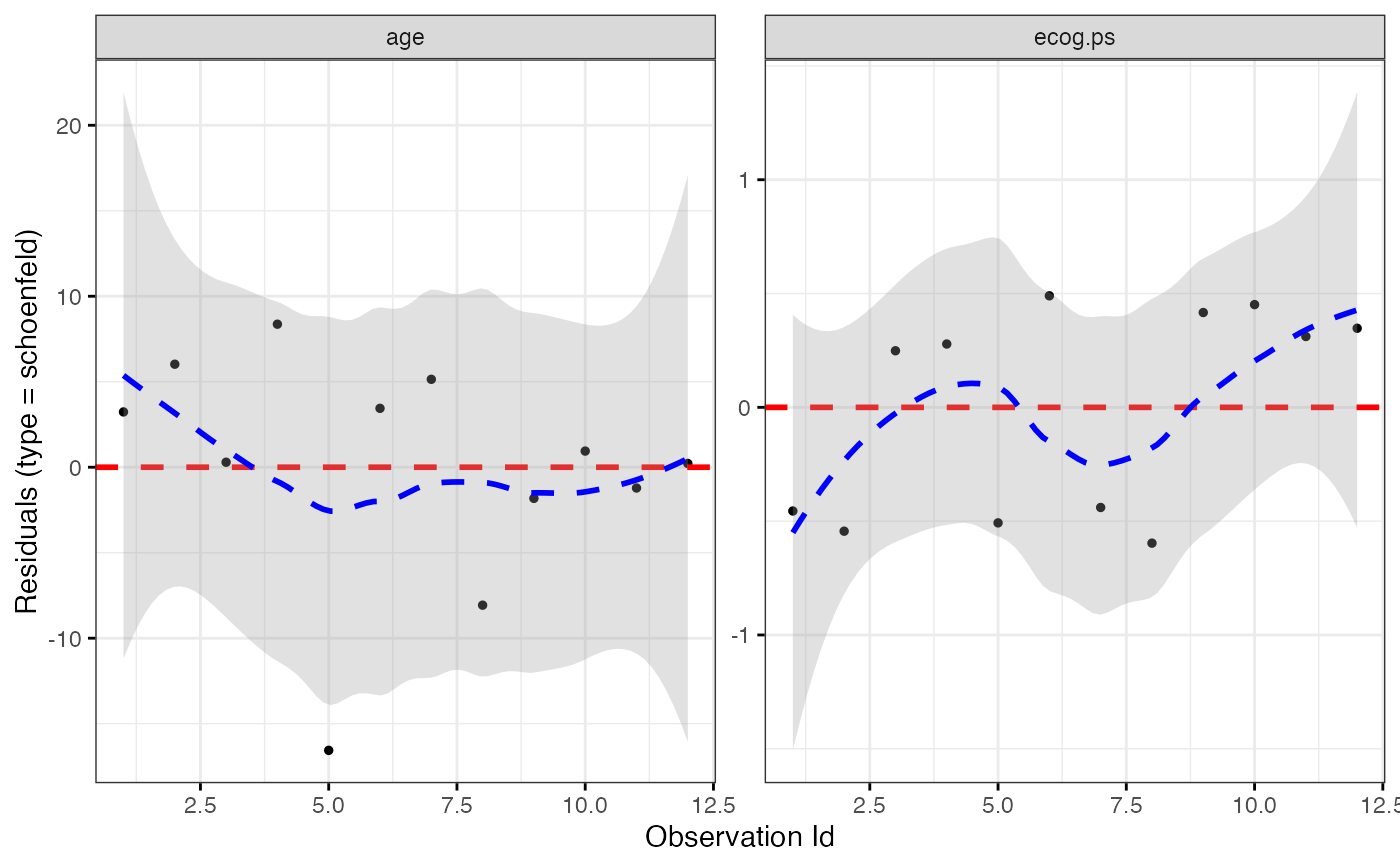ggcoxdiagnostics(coxph.fit2, type = "scaledsch", ox.scale = "time")#> geom_smooth() using formula 'y ~ x'